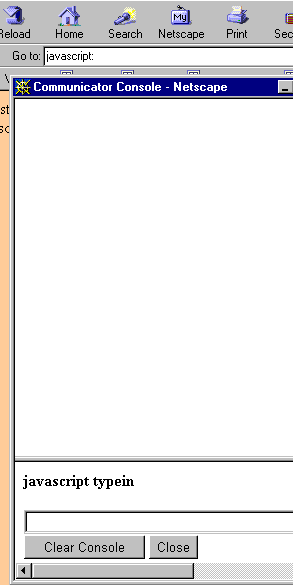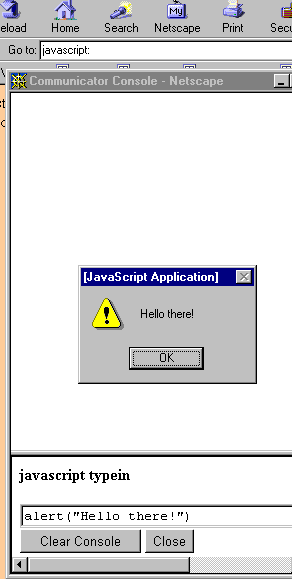The address for this web page is:   `www.crosswinds.net/~fishcaro/day_J2_javascript_basics.htm`
On to the next lesson!
Back to the COURSE SYLLABUS

# J2. JAVASCRIPT BASICS

INDEX CARD #J2:

## BASIC STUFF (J2a)

Is JavaScript case-sensitive? That is, when you type JavaScript code, does it matter whether you type "while" or "While" or "WHILE"? YES! It matters! JavaScript IS case-sensitive. Since HTML is not case-sensitive, you might not be used to typing things just-so, but you'll get used to it. As you study the following lessons, pay attention to which letters are capitalized and which aren't.

Can whitespace be used freely in JavaScript coding? JavaScript ignores most spaces, tabs, and newlines in programs, so you are free to format and indent your programs in neat, consistent, and easy-to-read ways. Pay attention to how programs are "laid out" in the lessons that follow, to begin to develop good programming style.

How can you tell when one statement ends in JavaScript, and another begins? Simple statements in JavaScript are generally followed by semicolons (;) . You can put more than one statement on the same line. Thus, you could write, on two separate lines:
``` a=3; b=3; ```
Or, you could combine the two statements on the same line, like this:
`a=3; b=3;`

There are several ways to insert comments in JavaScript programs:
• Any text between   //   and the end of a line is treated as a comment and ignored by JavaScript. Here's an example:
`// This is a single-line comment.`
• Any text (over one or more lines) between   /*   and   */   is treated as a comment. Here's an example which exhibits a nice style that makes a multi-line comment stand out:
```/* This comment * spans * more than * one line. */ ```

## HIDING JAVASCRIPT from old browsers (J2c)

Browsers that support JavaScript execute stuff in the SCRIPT container.
Some browsers that don't support JavaScript do, however, recognize the SCRIPT tag, and ignore everything inside, as they should.
However, some old browsers don't even recognize the SCRIPT tag: they ignore this tag, and treat all the Javascript inside as HTML text to be displayed, so your JavaScript code can end up being formatted into garbage paragraphs and displayed on your web page!
Here's a way to prevent this from happening. The reason it works is discussed on the next card, (J2d). The lines below are numbered for easy reference.
```line 1 <SCRIPT LANGUAGE="JavaScript"> line 2 <!-- Begin HTML comment that hides the script. line 3 all JavaScript statements go here line 4 // End HTML comment that hides the script. --> line 5 </SCRIPT> ```

## HOW DOES THIS HIDE THE CODE? (J2d)

There are two key ideas that make this "JavaScript-hiding-from-old-browser" technique work:
Javascript recognizes the HTML comment opening sequence, treating it as a single-line comment:  `<!--`
However, Javascript does not recognize the HTML comment closing sequence:  `-->`
So, here's what happens:
Browsers that don't understand the SCRIPT tags simply ignore lines 1 and 5. But, they'll also ignore lines 2, 3, and 4, because they're contained inside the HTML comment scheme: `<!--  -->`

But what about browsers that do support JavaScript? They recognize line 1, and know a script is beginning. They ignore line 2, because it is a one-line comment in JavaScript! Then they process line 3 (which represents all the JavaScript code). They ignore line 4, because it's a one-line comment. They see that the script is finished on line 5.
Very clever!

## PLAYING WITH JAVASCRIPT (J2e)

In Netscape Navigator (but not in Internet Explorer), if you type in the URL
`javascript:`
by itself, a JavaScript interpreter screen appears, as shown below this card (on the left). Then, you can type in JavaScript code, and press ENTER to see the code executed, as shown below this card (on the right).

This provides an easy way to play with JavaScript code!

In the Worksheet for this lesson, you'll use the Javascript Interpreter screen to explore arithmetic with numbers in Javascript, by typing in things like this:

``` x = 2+3; document.write("the value of x is " + x + "<BR>");```

Numbers will be discussed in more detail in future lessons; this is just a preview of coming attractions.

 JJAVASCRIPT INTERPRETER SCREEN:EXECUTING SOME CODE:WORKSHEET #J2:
(WJ2.1) Using Netscape, bring up a JavaScript Interpreter Screen, as discussed in (J2e). Type in the following lines, and observe the results. Don't worry about the "true" that comes up; this will be discussed later on.

1. `x = 2+3; document.write("the value of x is " + x + "<BR>");`
As expected, "+" is used for addition.
2. `x = 2-3; document.write("the value of x is " + x + "<BR>");`
As expected, "-" is used for subtraction.
3. `x = 2*3; document.write("the value of x is " + x + "<BR>");`
As expected, "*" is used for multiplication.
4. `x = 2/3; document.write("the value of x is " + x + "<BR>");`
As expected, "/" is used for division.
5. `x = Math.E; document.write("the value of x is " + x + "<BR>");`
This is the constant e, the base of the natural logarithm.
6. `x = Math.PI; document.write("the value of x is " + x + "<BR>");`
This is the constant pi, the ratio of the circumference to diameter in any circle.
7. `x = Math.abs(-2); document.write("the value of x is " + x + "<BR>");`
Math.abs(x) gives the absolute value of x.
8. `x = Math.ceil(2.3); document.write("the value of x is " + x + "<BR>");`
Math.ceil(x) rounds UP; it gives the closest integer greater than or equal to x.
9. `x = Math.floor(2.3); document.write("the value of x is " + x + "<BR>");`
Math.floor(x) rounds DOWN; it gives the closest integer that is less than or equal to x.

10. `x = Math.max(2,3); document.write("the value of x is " + x + "<BR>");`
Math.max(x,y) gives the greater of x and y.
11. `x = Math.min(2,3); document.write("the value of x is " + x + "<BR>");`
Math.min(x,y) gives the lesser of x and y.
12. `x = Math.pow(2,3); document.write("the value of x is " + x + "<BR>");`
Math.pow(x,y) computes x to the power of y.
13. `x = Math.random(); document.write("the value of x is " + x + "<BR>");`
Math.random() returns a randomly-generated number between 0.0 and 1.0 . (Use this one several times, and see the different numbers that appear!)
14. `x = Math.round(2.4); document.write("the value of x is " + x + "<BR>");`
Math.round(x) rounds x to the nearest integer.
15. `x = Math.sqrt(9); document.write("the value of x is " + x + "<BR>");`
Math.sqrt(x) gives the square root of x.
ASSIGNMENT #J2:
There's a good online tutorial on JavaScript, which you'll start in this lesson, and continue in future lessons. Please go to:
http://www.pageresource.com/jscript/index2.htm
Work through the following lessons, and do the additional questions/exercises:
• (AJ2.1) What you need to use JavaScript
• (AJ2.2) Basic Recognition of a JavaScript
1. How many `<SCRIPT>` tags can you have in an HTML document?
2. Inside a `<SCRIPT>` container tag, what is the attribute/value pair that lets you know that this is a JavaScript script?
3. If you're defining a JavaScript function (more in a future section!) then where should the `<SCRIPT>` container go?
• (AJ2.3) onMouseover: Your first Script
1. Does all JavaScript code HAVE to go inside a `<SCRIPT>`container?
2. WITHOUT LOOKING, be able to write the code that will say "Hi there! Do you like this site?" in the browser's status bar when you have your mouse over a link (your choice for the link HREF). The message should disappear when you move your mouse OFF the anchor. Test it in both Internet Explorer and Netscape Navigator.
3. Try typing "onMouseover" as "ONMOUSEOVER"; does it work?
4. Trying typing "window.status" as "Window.status". Does it work?
5. Switch the order of the "onMouseover" and "onMouseout" attribute/value pairs. Does it still work?
6. In your source code, try putting some carriage returns in various places. Where is it okay? Where does it NOT work? Comment.
On to the next lesson!
Back to the COURSE SYLLABUS